# Trend Is Not Your Only Friend

#### 26 July 2017 / Dr Ewan Kirk and Dr Chris Longworth

Simple rules on macro assets can create very attractive returns. We present a simple trend system and a simple carry system in which the combination of the two return streams appears very attractive.

### Summary

Trend following in one form or another has been an investment style for decades. Surprisingly to us, there is still a considerable amount of mystery surrounding how trend works and the important issues to consider when designing or selecting trend models.

Furthermore, although time series momentum dominates the systematic macro space there are many other return sources which are both persistent and diversifying. Often these are called “carry” or “value” or “risk-premia”. Of these terms, “carry” probably captures the essence of the return stream best and we will refer to the source of return as Carry.

In this piece, we are going to go through the construction of simple theoretical Trend and Carry models in some detail. The very simplicity of the models will help to clarify how the return sources work.

There are many implementation issues which mean that the simple theoretical models that will be presented here work poorly in the real world. These practical issues can be largely solved by more sophisticated model and portfolio construction, but our purpose here is to show that there are multiple source of return in the macro space.

As we shall see, the combination of both Trend and Carry considerably outperforms Trend alone.

### Assets and Scalification

We are going to be concerned with trading macro assets: Commodities, Interest Rates, Equity Indices and FX. Cash equities – as opposed to equity indices – are a very different micro asset set with different sources of return.

The choice of asset set is something that we could potentially vary, but in the macro space there is a natural set which includes most of the liquid futures and FX markets. The one that we will be using here is the same as that used in the SG Trend Indicator and are listed in this 2010 research paper. In the following the choice of asset set isn’t critical and as long as it is a reasonably diversified set of 50+ futures and forwards, all the results below hold.

The main advantage of trading a macro asset set is the sheer diversity of assets. The EURUSD exchange rate is very different from the price of oil or the Japanese stock markets. Although a tech stock like Google is somewhat different from a consumer stock like Unilever, the general characteristics of the returns are broadly similar and the price of the stock is primarily driven by “the market”.

This cannot be said of the futures markets. For example, here are the price series of two futures contracts on the CME: The S&P500 “e-Mini” future and the US two-year bond future.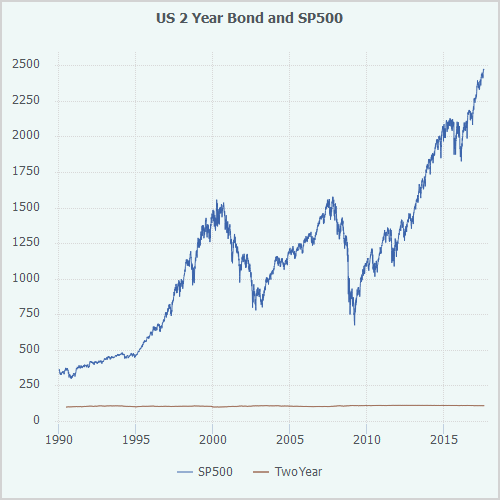It’s pretty clear from Figure 1 that these two contracts are very different, not just in price but also in how variable they are. If we want to model assets, it’s going to be easier if we can “normalise” them in some way.

The first thing one does is to work in return space. One cares about the percentage return on an asset, not the absolute price change.

We define the returns of an asset with price $p$ from day $t-1$ to day $t$ as

\begin{equation} r_{t} =\frac{ p_{t} - p_{t-1}}{p_{t-1}} \label{eq:returndef} \end{equation}

There are some gnarly details involved in trading futures contracts which make calculating returns on futures contracts more difficult. When do you roll the contracts and how do you adjust the returns of the series when you roll them? Whilst we are not ignoring them in this post, we are relegating them to footnotes to maintain some flow in the argument we are developing.

Having done the roll adjustment and rolled the series correctly, we can now chart the returns.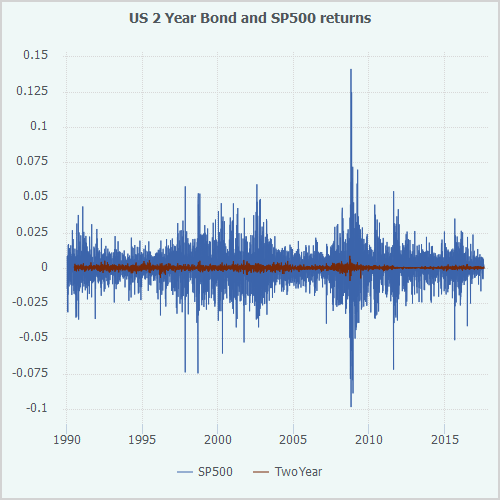There is clearly still a significant difference in the characteristics of the returns. The S&P 500 future has huge moves compared to the 2-year bond future. It is more volatile. Attempting to create a trading rule which can appropriately scale and risk manage the positions on not just the S&P 500 and the 2-year bond, but on the very diverse assets in our asset set is a challenge when the volatilities differ so wildly. To handle this problem, it is time to introduce the concept of volatility scaling or, as we call it at Cantab, scalification. This allows us to retain the diversification benefits while making the modelling of the assets much easier.

Volatility is defined as the annualised standard deviation of the returns of an asset. One standard way to calculate the volatility $\sigma_W$ of an asset over a window $W$ at time $t$ is

\begin{equation} \sigma_t^W = \sqrt{\frac{256}{W}\sum_{i=1}^{W}{(r_{t-i}-\mu)^2}} \label{eq:voldef} \end{equation}

where $\mu$ is the mean of the returns over the window. The number 256 converts the standard deviation into an annualised number by assuming that there are 256 business days in a year.

The volatility depends on the size of the window. Long windows will tend to provide more stable estimates, whereas short windows are more reactive. We can also use different techniques to calculate volatility. For example, we could exponentially weight the returns making more recent returns more “important” or we could explore the wilds of autoregressive conditional heteroskedasticity target="_blank". For our purposes, we will continue to use the simple definition above and, unless explicitly stated otherwise, we will use a window of 100 days.

We will define a function $S$ – the scalification function – as

\begin{equation} S_t(x) = x\frac{0.1}{\sigma_{t-1}} \label{eq:scalifydef} \end{equation}

This function converts the returns of an asset into returns which have an annualised volatility of 0.1 (or 10% as it is normally called). We define scalified returns for an asset as

\begin{equation} \hat{r}_t = S_t(r_t) = r_t\frac{0.1}{\sigma_{t-1}} \label{eq:scalifiedreturns} \end{equation}

Let’s now look at the scalified returns of our two sample assets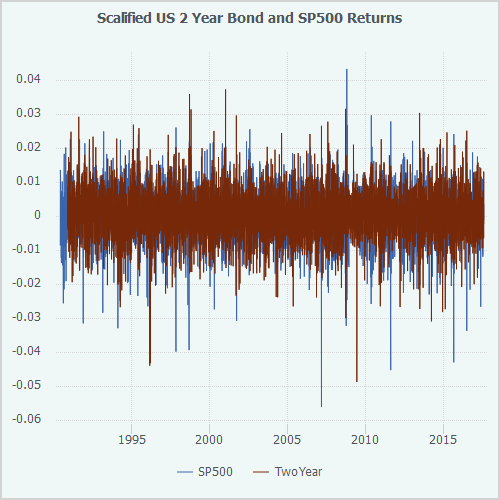These look a lot more similar. It’s sometimes hard to see these similarities in returns graphs so we can accumulate up these returns to create a simulated price series for these scalified assets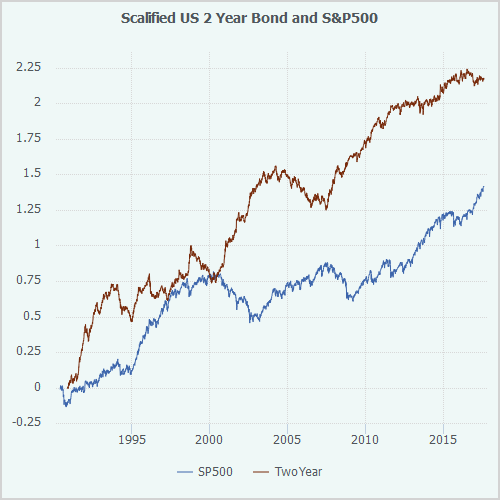Our models are going to signal on these scalified assets. Of course, these assets don’t actually exist and therefore whatever position we want to have in scalified assets has to be converted back into a position in the real asset. If our scalification model works well (and it generally does), the notional position that we have in USD for each asset will have a 10% volatility.

### Signal Generation

Now that we have our data on the same scale, we can start to think about how we generate the decisions to be long or short.

Signal generation is about building a rule which transforms some market or non-market inputs into a desired position on the scalified asset. Depending on the type of return one is hoping to harvest, the rule for transforming the inputs into a desired position will be different. We are going to look at two such rules: Trend following and Carry trading.

### Trend Following

Trend following has a long history. At its heart, it encapsulates the view or belief that “things which have been going up for a bit tend to keep going up and things that have been going down for a bit tend to keep going down”. Although there are many hypotheses purporting to “explain” Trend, we will just make the statement that “trend following has worked in the past and that’s a good enough reason to do it in the future."

#### Generating the Signal

There are literally hundreds of ways of filtering time series to create a Trend signal, but we are going to use the simplest we can think of: averaging the returns over some “medium-term” window. Definitions are somewhat fluid in this space, but let us make a decision to define “medium-term” as “around 4 or 5 months” or 100 days.

Formally, our signal $s$ on an asset with scalified returns $\hat{r}$ is

\begin{equation} s = \text{sign}\Bigg({\frac{1}{W}\sum_{\tau=t-W}^{t}{{\hat{r}}^i_\tau}}\Bigg) \end{equation}

where $W$ is the window length (100 in this case). The sign function returns +1 if the argument is positive and -1 if the argument is negative.

In non-mathematical notation, this says “buy a market if it is above its 100-day moving average and sell if it’s below."

#### Calculating the Results

We now have everything we need to construct a simple 100-day moving average model on a basket of more than 60 futures which have been scalified. There’s just one final detail. Once we have our positions on all the scalified assets, how do we combine them?

In our case, we are going to simply add together all the asset positions, but this has a very obvious problem. If we’re adding together lots of 10% volatility assets, we are going to get a higher volatility than 10%. Also, given that the correlation between assets is variable, the volatility of the portfolio won’t be constant.

This is not ideal in an investment strategy. To solve this problem in a simple way, we construct the portfolio by adding together all the positions and then performing the scalification process one more time. We estimate the 100-day volatility of the entire portfolio using the same technique as above and then scale the positions uniformly to make this 10%.

And here are the results: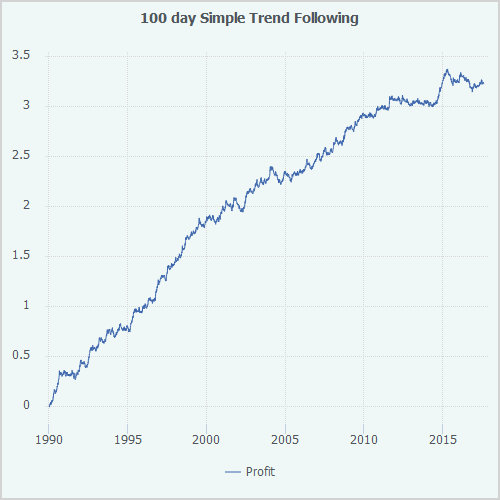Annualised ReturnAnnualised VolSharpe Ratio
Simple Trend11.4510.351.11

#### Discussion

This is rather good. A relatively simple rule with a relatively simple portfolio algorithm has produced a 1.11 Sharpe ratio gross of fees and trading costs. Our simple trend model also has the same gross characteristics as “real” trend-following funds. A biggish drawdown in 2004, long flat period from 2011 to mid-2014 and a very strong end to 2014.

The fact that such a simple model can generate these risk-adjusted returns is very encouraging. Obviously we could make this model much more sophisticated but one of the rules of thumb of systematic modelling is that if one can’t generate profits with relatively simple models, then a more sophisticated model which does generate profits should be treated with extra scepticism.

Trend is a well-known source of return and it powers the majority of the returns in the Systematic Global Macro space. However, there are other systematic rules that can be applied to macro assets which can also generate attractive returns.

### Carry

Carry is another concept with a long and storied history. Sometimes unfairly characterised by the phrase “picking up nickels in front of a steam roller”, Carry is (simply) the return that you get from holding a higher yielding asset and funding it with a lower yielding asset. Carry can also be characterised by statements about value or price: it is the return that you get from buying a cheaper (more valuable) asset and selling a more expensive (less valuable) asset. Carry is ubiquitous across finance and can often be harvested using simple rules.

The definition of “higher yielding” or “more valuable” differs between asset classes.. Our basic model for carry is that we have an indicator $I$ which measures whether or not an asset $A$ has a positive or negative expected carry. For each asset, our scalified position in that asset is

\begin{equation} P_A = \text{sign}(I_A) \end{equation}

In each asset group we will add up the scalified positions and then retarget that portfolio to a 10% volatility.

#### FX

Foreign Exchange carry is probably the most famous of the carry trades. Buy a high-yielding currency, sell a low-yielding currency. Practised by everybody from famous macro traders of the Nineties to the apocryphal Mrs Watanabe buying Australian Dollar with her Japanese Yen.

We are going to use a fairly standard definition of FX carry in our simple carry strategy. We will use the one-month money market rates for each of the G10 currencies. For each currency, we subtract the USD one-month money market rate from the relevant currency and then rank order these from highest to lowest. We will then go long one (scalified) unit of the top three interest rate differential currencies and short one (scalified) unit of the bottom three interest rate differential currencies. We compare the money market rates at the end of each day and if the currencies in the top three or bottom three have changed, we will change our position the following day.

In a very similar way to our final portfolio construction for Simple Trend above, we apply a further level of scalification to normalise the volatility of the FX Carry portfolio to 10%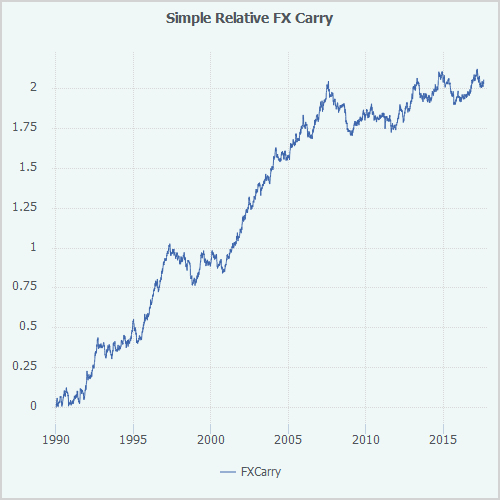Annualised ReturnAnnualised VolSharpe Ratio
Simple FX Carry7.2710.330.70

While a Sharpe ratio of 0.70 isn’t as good as the simple trend result, it is still respectable. It has the expected characteristics of FX carry, namely very good performance from 2000 to 2007 coming from funding high-yielding currencies like AUD with JPY. It also shows the “carry unwind” in 2008 and in 1998 as a result of flight to quality during crises.

#### Interest rates

For rates, the simplest definition of carry or value is relative yield. Our indicator, $I$, will be the difference between the 2-year bond yield and the 10-year bond yield. If the yield curve is upwardly sloping, we will go long the 10-year bond; if it is downward sloping we will go short. We use those markets where there are reasonably liquid futures contracts, namely US, Europe, UK, Japan, Canada and Australia. Given that yield curves have been upward sloping most of the time since 1990 and that bonds have had a pretty good run in the past 25 years, we would expect this to have performed well.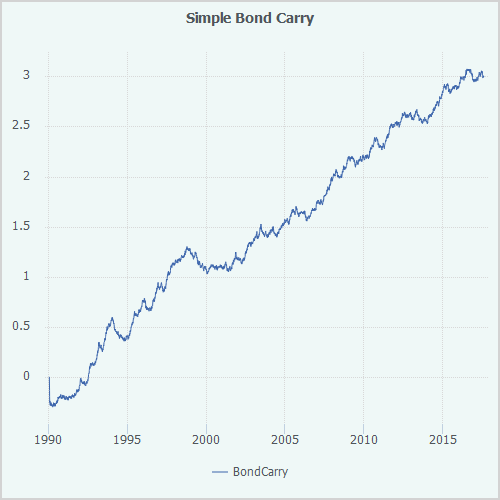Annualised ReturnAnnualised VolSharpe Ratio
Simple Bond Carry10.6310.631.00

We should probably be a little sceptical that this performance can be maintained in the future…

#### Equities

We are going to define a very simple carry or value indicator for equities. If the implied dividend yield on the equity index is greater than the local one-month money market rate, we will be long that index. If it’s less, we will be short the index. In essence, we are saying that if equity dividends are less than cash returns, you should just invest in cash and go short equities.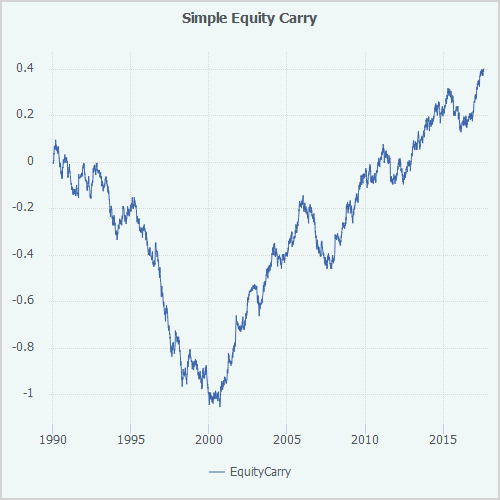Annualised ReturnAnnualised VolSharpe Ratio
Simple Equity Carry1.4110.470.14

As equities prices soared from 1995 to 2000 and interest rates were relatively high, the short position in equity indices performed exceptionally badly. We recovered the losses during the dot com bust and in the low interest rate environment after the financial crisis, but overall the performance is poor.

It is naturally quite tempting to say “ah, carry doesn’t work on equities”, but cherry picking asset classes is dangerous. Our hypothesis is that all assets have a carry return and that we will “blindly” execute the resultant trades. This blog is about creating the simplest possible implementations of trend and carry. We can be smarter later.

#### Commodity Carry

In the 1990s, there was an explosion of “Commodity Indices”. These were designed to be a passive long-only investment in commodities. Complex and ingenious weighting schemes were devised to mimic the market capitalisation weighting of equity indices. For almost all commodities, it is impractical and uneconomic to actually hold the commodities physically so they are implemented by holding a long position in a commodity future. The return driver for these indices was not that the price of commodities goes up (in general it goes down over time), but that holding a deferred futures contract until close to expiry and then rolling it to the next future generates positive returns. Or at least it did for markets which are in backwardation. This is where the price of the commodity for prompt delivery is higher than the price for a longer dated future delivery. Energy contracts were often in this state in the Nineties, and - since energy was a big component of most commodity indices - this made them very attractive. In order to construct a true long-only “index”, these products also had to be long those markets which were in contango (prompt delivery price is less than deferred delivery price). Sadly, holding a long rolling position in a commodity which is in contango does not work out well.

We are not attempting to be an index however. Our commodity carry indicator will be the difference between a front month contract and a deferred contract. If this is positive, we will go long the commodity. If it is negative we will go short the commodity.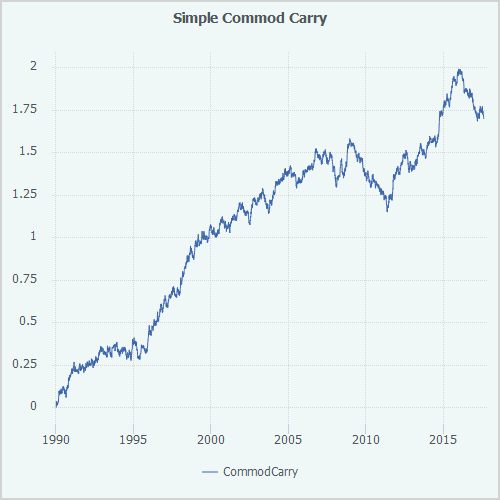Annualised ReturnAnnualised VolSharpe Ratio
Simple Commodity Carry6.0210.210.59

This has a gross Sharpe ratio of 0.59 which is once again surprisingly good given the simplicity of the model.

#### Putting it all together

In the spirit of keeping everything as simple as possible we are going to add together the four carry portfolios above. The combined portfolio is going to be scalified to a 10% volatility.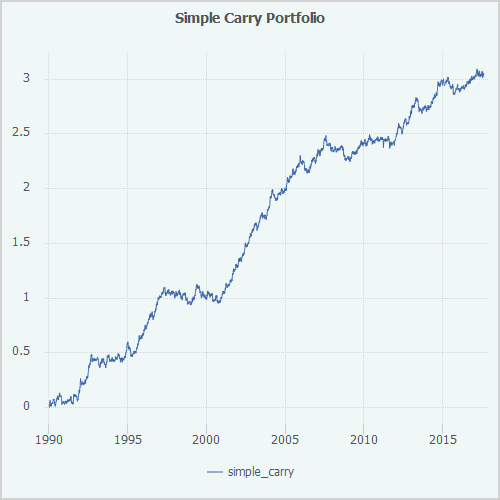Annualised ReturnAnnualised VolSharpe Ratio
Simple Carry10.8110.341.05

Once again, by applying some simple mechanical rules to a set of assets, we have managed to create a strategy which has a very respectable Sharpe ratio of 1.05.

It is surprising that Carry is less well-known and more infrequently implemented than Trend. The performance is similar, and, if correctly implemented, could have real benefits for investors’ portfolios.

### Free Lunch

At this stage, it becomes natural to think about the combination of Trend and Carry. Unless they are 100% correlated, there will be diversification benefits to running both simple strategies in one “Macro” portfolio.

If we calculate the correlation between each of the components and also their correlation to traditional assets we find the following

CorrelationTrendCarryEquitiesBonds
Trend1.000.13-0.120.18
Carry0.131.000.160.08
Equities-0.120.161.00-0.08
Bonds0.180.08-0.081.00

Trend and Carry have a statistically insignificant correlation to each other and a negligable correlation to the traditional markets. This suggests two things:

• Firstly the combination of Trend and Carry should be better than each individual one and
• Both of these return sources should be very valuable in a more traditional portfolio of equities and bonds.

We place the two separate return streams of Trend and Carry into a portfolio with 50% weight on each and rescale that portfolio using our scalification algorithm one final time.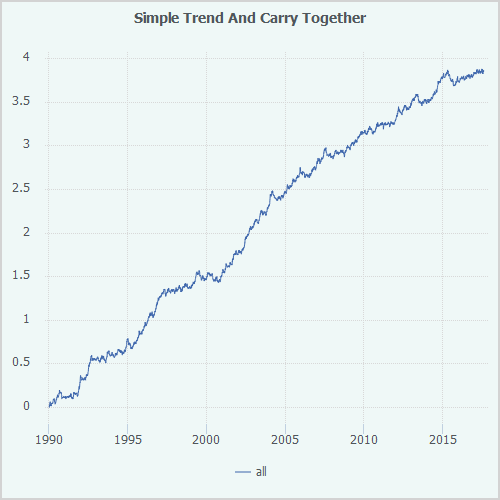Annualised ReturnAnnualised VolSharpe Ratio
Trend and Carry13.6810.351.32

With a set of relatively simple rules, we have reached a Sharpe ratio of 1.32! This is statistically significant and an impressive level to have reached with such simple rules.

It is also interesting to look at the individual years for the Trend and Carry portfolio versus Trend alone.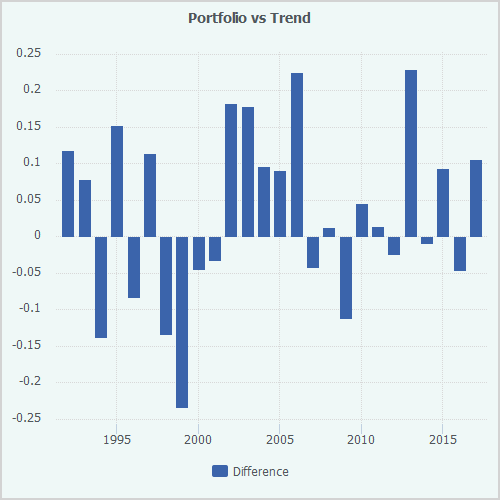Figure 12 is possibly somewhat surprising. Despite the impressive increase in Sharpe ratio, the portfolio doesn’t always outperform the trend model. Indeed, this will always be the case when one is combining two return streams. There will always be a period where the combination underperforms as our portfolio does in the late Nineties. But, “life is long” and in particular, one’s investment horizons should be long. Trusting in the value of diversification is one of those things where a long timeframe is essential. In the period from 1997 to 2000, the portfolio underperforms by a total of 43% despite outperforming by more than 2% per annum on average. In recent times from 2012 to 2016, the portfolio has outperformed Trend by 37%.

The question of whether or not one can “time” Trend and Carry in such a way as to ensure perpetual outperformance could be the subject of another piece, but suffice it to say that the answer is “no, you can’t”.

### Conclusions

We have constructed two very simple systematic models - one as a proxy for Trend and one as a proxy for Carry. The full specifications of the models have been presented and, in principle, any reader with access to an Excel spreadsheet or another simple analytic system could reproduce this work. We have shown that not only are both Trend and Carry valuable sources of return uncorrelated to traditional assets, but the combination of the two outperforms each individual return stream.

There are obviously some caveats and concerns here:

• These are all backtests. There is no guarantee that Trend or Carry will continue to perform in the same way as they have in the past. However, given the persistence of these return streams over decades, we can probably be reasonably confident that they will persist.
• These are simple models. Our belief is that if one can construct simple models and rules which produce attractive returns then more subtle and complex rules have a higher probability of working. The models above have many flaws. For example, it’s very difficult to believe that anybody would implement our naïve version of equity carry. Losing money in a straight line for 10 years on a strategy is unlikely to be a recipe for long-term career success or investor loyalty. There are many better ways of capturing the concept of “equity carry”. However, that being said, as one adds complexity, one can add fragility or, to use a phrase from the machine learning community, “poor generalisation”. Care is needed.
• Most importantly, we have completely ignored any costs of trading and liquidity constraints. We have assumed that one can trade instantaneously at the mid in any size when required to. This is an exceptionally unreasonable assumption. The results for simple models look very different in the presence of costs whereas the more sophisticated models will minimise trading costs. Indeed, cost minimisation is often a feature of more sophisticated modelling.

It is not our purpose here to propose these models as investable. As we said, they are almost laughably simple and naïve. In the real world, they would be destroyed by trading costs. Also, once the real world starts to encroach, one has to deal with all sort of implementation issues such as markets not being open at the same time, liquidity constraints, exchange limits, execution costs, software reliability. One’s spreadsheet (or ZX81) is going to struggle mightily when faced with the blizzard of real-time data and the execution and liquidity challenges of the markets.

We will postpone covering these challenges until a future date. What we have shown is that alongside the well-known time series momentum return stream known as Trend, there is another equally valuable return stream called Carry and combining the two results in a very attractive and valuable return stream.

The trend isn’t your only friend.